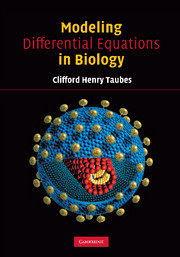Modelling Differential Equations in Biology: Given that a college level life science student will take only one additional calculus course after learning its very basics, what material should such a course cover? This book answers that question. It is based on a very successful one-semester course taught at Harvard and aims to teach students in the life sciences understanding the use of differential equations. It is enriched with illustrative examples from real papers. Necessary notions from linear algebra and partial differential equations are introduced as and when needed, and in the context of applications. Drawing on a very successful one-semester course at Harvard, this text aims to teach students in the life sciences how to use differential equations. It is enriched with illustrative examples from real papers. Necessary notions from mathematics are introduced as and when needed, and in the context of applications. Aimed at biologists wishing to understand mathematical modelling rather than just learning math methods.